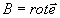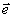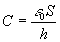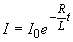content

### Felix Gorbatsewich - The Ether and Universe

8. Strain of the ether medium in electric and magnetic fields

The influence of an electric current on the magnetic needle behaviour was first found by Oersted in 1820 . Faraday observed the electroinduction phenomenon . Later it was shown that equipotential lines of the magnetic field round a conductor with current are concentric circles. Here is how Pointing described the rise of lines of force round a conductor with current: "When the strength of the electric current that flows in a direct conductor increases gradually from zero, the ambient space is being filled with magnetic lines of force that have a shape of circles arranged around the conductor's axis. “….”These lines of force get to their places moving outside from the conductor in such a way that the magnetic field grows since the conductor constantly emits lines of force which expand and travel just as ripples caused by a stone thrown in still water”. .

Then an electromotive force (EMF) of self-induction was found. According to Lents's law , inductive currents are always directed in such a way that their proper field counteracts the changes of the field that cause them. EMF of self-induction appears when turning off an electric circuit. Since the magnetic field around the conductor with current disappears when turning off the circuit, one may presume that the EMF of self-induction is formed due to transformation of magnetic field energy into electric current.

According to our concept, the self-induction mechanism is the following. At the initial stage of switching on an electric circuit the electric current cannot reach its maximum value at once, since part of its energy is spent on formation of a magnetic field in the ether medium around the conductor (here we consider the conductor placed in the evacuated space). The magnetic field cannot rise instantly, since electromagnetic disturbances in the vacuum propagate with the final velocity - velocity of light C. In principle, the magnetic field of the conductor with current extends over indefinitely great distances.

The magnetic field is maintained all the time while the current flows in the conductor, Fig. 9 and 15. When turning off an electric circuit the magnetic field energy stored in the ether medium is given back to the conductor as a self-induction current. Quite apparently, during switching on a circuit, part of the electric energy is elastically stored as a magnetic field around the conductor. During switching off it elastically comes back to the circuit as EMF self-induction. Some part of the energy is irreversibly spent for electromagnetic radiation both during switching the current on and off.

Thus, the ether medium or vacuum around the conductor plays the role of an elastic element storing energy. Around the conductor with current a torsion strain (a kind of a shearing strain) described by MacCullagh's tensor appears (12).

It is rather difficult to think up other mechanisms that might adequately explain both the phenomenon of self-induction and other effects observed in experiments. Theoretically, one may assume the existence of single material carriers of a magnetic field that are able to move only along closed trajectories. P. Dirak made attempts to provide theoretical grounds to the presence of individual carriers that were called monopoles [55, 60]. Naturally, such monopoles can move not only along closed trajectories. If the single carriers of a magnetic field had existed they might be accumulated at the poles similar to electric charges and would have been of a static nature. In this case they would have been easily detected experimentally.

One might imagine magnetic monopoles as waves moving around a conductor with current. But in this case also a contradiction arises, i.e. the allowed velocity of electromagnetic waves in the ether is only the velocity of light С that is close, as is known, to a constant. Thus, the magnetic wave that moves around a conductor with current with various velocities depending on the distance to the conductor, cannot exist around this conductor. Previously Yu.K. Sakharov  noted that the law of conservation of energy forbids the existence of a magnetic monopole.

A substantiation of a possible existence of gaseous or liquid ether encounters more principle contradictions than its quasi-solid model. It is extremely difficult to imagine and mathematically simulate closed streams of gas or liquid particles without formation of local swirls, instabilities, different forms of laminar, turbulent and other kinds of movement. The behaviour of real gases follows the laws of thermodynamics. As is known, it is just the motion instability that is typical of the flows of real liquids including superfluid ones. Observations on the magnet component of radio waves of various frequency in the interplanetary space showed that its velocity as well as the velocity of light C is near to constant . This suggests a substantial homogeneity of the ether medium and points to a low probability of dynamic instability of various types existing in this medium.

A movement in a vortex formation (a set of medium particles embedded into each other and moving along circular paths) should be with a different velocity in relation to the distance to the centre of rotation. The laws of equality of angular momentum and medium continuity should be followed for every ring embedded in the vortex. On the vortex periphery the particle circular velocity is lower, as the particles move to the centre, the velocity increases. As observations show, at some distance from the centre, the circular velocity acquires the maximum value. Father to the vortex centre the circular velocity of particles decreases. This phenomenon can be explained by weakening of the centrifugal force action on inertial particles (i.e. massive particles). The velocity decrease in the centre of the whirl is most conspicuous during atmospheric cyclones, hurricane, typhoon, tornado etc. Fig. 16 gives the profile of the air speed in an atmospheric typhoon that corroborates this phenomenon. The velocity profile of this natural phenomenon was registered in Manila on 20 October, 1882 . The total time of the typhoon passage through the town was about 12 hours. During 5 hours the window speed V reached 60 m/s. Then it fell sharply virtually to zero in the tornado centre. After passing the centre, the wind speed again reached its maximum.

Experiments show that around a conductor with current, the gradient of decrease in the magnetic field stress is directed away from the conductor and its function is monotonous [57, 67]. In this case, as is shown above, the ether medium has a specific mass (see Sect. 6) and the processes of magnetic field formation around the conductor with current are inertial. If the magnetic field had been really vortex then we would have observed a rather weak magnetic field near the surface of the conductor with direct current. At some distance it would have acquired the maximum value. Then, as the magnetic field moved away from the conductor, it would have decreased monotonously. Thus, the known Maxwell's equation(is elastic displacement) cannot be brought into correlation with the vortex motion of an inertial medium. The above Maxwell's equation suggests a monotonous decrease of the magnetic flux from the linear current along the normal to this current.

As shown above, the distribution of velocity and power in the vortex motion of particles possessing inertia (mass) differs radically from that described by the Maxwell's equation. In fact, a monotonous decrease of the magnetic field value around the conductor with direct current begins directly at the conductor surface. These observations count in favour of MacCullag's hypothesis of quasisolid ether (see Sect. 5).

We should also notice that an imagination of a magnetic field as a vortex near, for instance, a permanent magnet suggests an availability of a dynamic motion. But the field of a permanent magnet is really constant. It does not reveal itself if there is no other magnet or moving conductor. After W. Thomson (see Sect. 2), a dynamic movement formed by the flow of hypothetical magnet particles near a cylindrical solenoid with current, would really draw the particles inside the solenoid. But then the flow of particles forming the magnet field would push those particles out of the solenoid. Carrying the particles out of the solenoid would occur, if for no other reason than the sluggishness (inertness) of their movement. Experiments show that ferromagnetic particles drawn into the solenoid remain in it. They remain in the area of the maximum stress of the magnetic field, i.e. where the maximum strain of the ether medium is observed.

From the above, it is most logical to imagine a magnetic field as a torsion (shear) strain of the ether medium. It has been strictly proved that magnetic lines of force are always closed. Equipotential lines of elastic shear strains are also always closed . In this case the so called medium continuity condition is met. It should be believed that the medium continuity condition is valid for ether as well.

Particles in the ether medium are rigidly bound, so they can move there only relative to each other forming strains of this medium. Rotary motions of one particles relative to others do not occur in the medium. If ether particles could spin, then the light passing through the field of a strong magnet would be polarized.

As shown above, in the ether medium only torsional and shearing strains, i.e. strains of a form are possible. Accordingly, in the strained ether medium, magnetic fields of different forms can exist. They reflect the strain form of the medium. Potential power in the ether medium is stored as a magnetic field and an electric charge field. Both these fields reflect different strains of the ether medium. Dynamic effects occurring in the ether medium will manifest themselves as displacement currents.

A torsion strain can be expressed by the equation describing a linearly decreasing strain with the increase in the distance from the linear conductor. As shown by W. Thomson and A. Zommerfeld, it can be fully described by the Maxwell's equation(see Sect. 5).

Imagine simple models of the ether medium strain in accordance with the concept of the quasi-solid ether. As shown above, when an electric and (or) magnetic field are superimposed, the ether medium particles will shift from their equilibrium position that they would occupy in the space-netlike structure in the unperturbed state. Consider a displacement pattern in the ether medium at the superposition of an electrostatic field on the medium, for instance, between the plates of a charged vacuum-processed capacitor. In this case the ether medium, Fig. 8, will undergo strain in the way shown in Fig. 17. In this case the ether medium's particle with the positive charge will move away from the positively charged plate. On the contrary, a negatively charged particle of the ether medium will be in contact with the positively charged plate.

Naturally, the diagram in Fig.17 (plane section) is greatly simplified since the charges, for instance, electrons will take places in the capacitor plate in accordance with the electron gas laws and the picture, on the whole, will be more complicated. Note that the displacement between the positive and negative particles of the ether medium in the capacitor field will be of some value z .

The capacitance C of the vacuum-processed capacitor can be calculated by formula :, (35)

where e 0 is the vacuum inductivity, S is the area of the capacitor plates, h is the distance between its parallel surfaces.

As follows from formula (35), the capacitance of the vacuum-processed plane capacitor is independent of the thickness of its plates and their conductivity, of the kind of material they have been made of etc. The value S/h involved in the formula refers only to the volume of the ether medium that is between the capacitor plates. If we deliver charge to the plates of a plane capacitor, Fig. 17, then in the S× h volume a strain of the ether medium (vacuum) will appear. The larger is the area of the capacitor plates, the greater is the volume of the strained vacuum. With the same charge at the capacitor plates, the shorter is the distance h, the greater is the electric intensity between the plates. Great electric intensity causes a great degree of the vacuum strain. Thus, it is exactly the ability of the ether medium to be strained that is responsible for the capacitance of a flat (as well as of all the other types of) capacitor.

Let us imagine how the strain of the ether medium occurs in a magnetic field. According to the above grounds, the ether medium in the magnetic field is strained by shear forces, every subsequent row of the medium being shifted from the preceding one in one and the same direction. Figure 18 gives a plane section of such a shear strain of the ether medium in a homogeneous magnetic field that will be observed at one side of the central part of a very long (quasi-infinite) solenoid. Note that in a homogeneous magnetic field every row is shifted from the preceding one by some value of strain x .

As is known , inductance L of a cylindrical solenoid (inductance coil) depends on the magnetic permeability of the medium m , its length l, the area of the cross section S and a number of coils N. For the solenoid that is in the vacuum, with the relation of the length l to the diameter of its coils d, l/d > 10, the value

L = m0×n2V, (36)

where m 0 is magnetic permeability of the vacuum, n = N/l is a number of coils per unit length, V = Sl is the solenoid volume.

As for a capacitor, the inductance of a solenoid is independent of the conductivity and type of the conductor material. In formula (36), attention is drawn to the peculiarity that the solenoid inductance is determined, besides other factors, by the volume V and the properties of the vacuum m 0 which is inside its coils. It is quite clear that if the volume V is equal to zero, the solenoid inductance will be also equal to zero. The increase in the number of coils n or in the number of currents per unit length of the solenoid, greatly increases the vacuum strain degree.

Experiments with solenoids allow one to demonstrate clearly such an important vacuum feature as its sluggishness. For instance, when the source of electromotive force (EMF) in the circuit with inductance L and resistance R is switched off, the current l will change according to the law :, (37)

where I0 is the current initial value before the circuit is switched off, t is time.

From formula (37) it follows that the greater is the inductance L and, accordingly, the greater space (vacuum) is occupied by the solenoid internal volume, the slower is the current decrease from the initial value in the circuit. On the one hand, when a circuit with current is switched off, the magnetic field in the solenoid and around it decreases and disappears. On the other hand, the decrease of the magnetic field occurs by the exponential law. It means that theoretically the field changing continues infinitely long. Since the velocity of electromagnetic disturbances in the ether medium the velocity of light, one may believe that when switching an electric circuit off (on), the change in the magnetic field covers indefinably great space with time. Accordingly, in this case the sluggishness of the ether medium is formed by the availability of physical, i.e. magnetic field and the final velocity of disturbances in the medium. Similarly, as inertial properties of the vacuum are displayed, discharging or charging of the capacitor, including a vacuum-processed one, takes place . Thus, sluggishness is an integral and essential feature of the vacuum (ether medium).

An analysis of Figs. 17 and 18 allows one to understand why there is a great difference in magnetic and electric forces acting on various materials. For instance, the attraction of two electrified bodies is rather small as compared with the force of attraction of a piece of iron to a magnet. Really, when the ether medium is strained by an electric field, Fig. 17, the second row of charges shifts from the first row by z . Such a shift occurs in every other row. The overall shift Ue of the neighbouring rows of the ether medium elements will be no more than z .

Another situation will be observed if we consider the total shift of the rows of the ether medium elements Um in a magnetic field, Fig. 18. Here the second row will shift from the first row by the value x . Every subsequent row will shift in the same direction and by the same value x . The overall strain of the ether medium for a number of rows n will be Um = x × n and will be proportional to the value of the magnetic field section under consideration. A comparison of Um and Ue shows that the magnetic force is n times greater than the electric one, all other things being equal. Accordingly, magnetic fields demonstrate much greater forces than electrostatic ones. Practice, including that in technical applications, corroborates that. This practice has been embodied in power electric motors, electromagnets and many other applications. Due to the fundamental difference in the action on the ether medium, electric fields cannot develop considerable forces. That is why up to now electrostatic power machinery has not found such a wide application as electromagnetic one.

The diagram in Fig. 19 allows explaining the interaction mechanism of two circulating currents (magnets) and strains of the ether medium produced by them. The Figure gives a part of a homogeneous magnetic field excited by two magnets (circulating currents). Such a magnetic field can be excited inside an infinitely long solenoid. This field represents the strained ether medium with an axial symmetry and a circular section. By convention the North magnetic pole N is directed to the convex part of the equipotential lines of this field, while the South pole S- to the concave lines. The arrows in the diagram point to the direction in which the ether medium particles will shift when the current is switched off in the solenoid. If we move the field of the upper solenoid closer to the field of the lower one, Fig. 19, they will undergo attraction due to the same direction of the strains of the solenoid fields.

Quite another picture will be observed if the like poles of the magnet fields face each other, Fig. 20. In this case due to the fact that the ether medium strains from each solenoid will have opposite directions, considerable repulsive forces will appear.

Observations show that when two magnetic cores approach each other, their unlike poles close up and form a unified magnet. When the like poles of magnets approach each other, a certain effort is required to keep them closed up. If this effort disappears, they will disconnect. In this case the position of one magnetic core relative to the other becomes unstable. The magnets will tend to occupy such a position at which they will create the ether medium strain with the same direction.

The suggested concept eliminates the magnetic field paradox that, up to now, has been called vortex everywhere in reference books and text-books [57, 67, 68]. In our opinion, that is wrong.

Thus, an electric and magnetic fields are different forms of shear (torsional) strains of the ether medium composed of particles with opposite charges. The ether model we have proposed complies with the principles of I. Newton, J. MacCullagh, J. Maxwell and W. Thomson's theories .

The concept of the ether medium presented above allows one to understand why moving electric and magnetic fields (as well a stationary one) in O. Lodge's experiments did not have a noticeable impact on the light velocity (see Sect. 2). The point is that electric and magnetic fields do not change the ether medium density, they only strain it (Fig. 17 and 18). The strains induced by a massive physical body decompact the ether medium (Fig. 12 and 13). A deviation and change of the light velocity near massive bodies (for instance, the sun) is a well established physical factor .

content